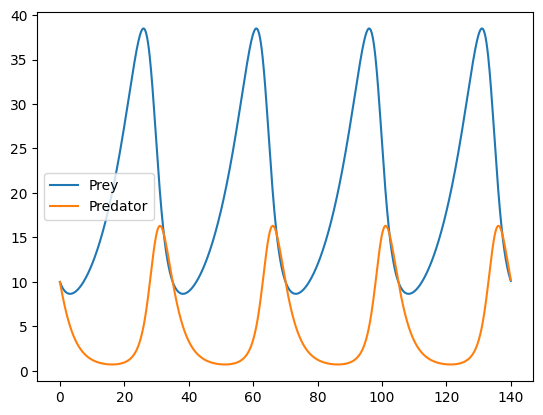# Coupled ODEs¤

This example demonstrates basic functionality for solving a system of coupled ODEs; in this the Lotka–Volterra equations.

This example is available as a Jupyter notebook here.

import jax.numpy as jnp
import matplotlib.pyplot as plt
from diffrax import diffeqsolve, ODETerm, SaveAt, Tsit5

def vector_field(t, y, args):
prey, predator = y
α, β, γ, δ = args
d_prey = α * prey - β * prey * predator
d_predator = -γ * predator + δ * prey * predator
d_y = d_prey, d_predator
return d_y

term = ODETerm(vector_field)
solver = Tsit5()
t0 = 0
t1 = 140
dt0 = 0.1
y0 = (10.0, 10.0)
args = (0.1, 0.02, 0.4, 0.02)
saveat = SaveAt(ts=jnp.linspace(t0, t1, 1000))
sol = diffeqsolve(term, solver, t0, t1, dt0, y0, args=args, saveat=saveat)

plt.plot(sol.ts, sol.ys, label="Prey")
plt.plot(sol.ts, sol.ys, label="Predator")
plt.legend()
plt.show()